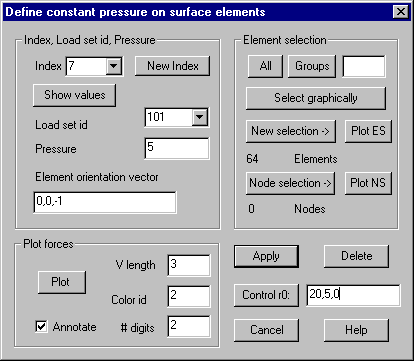Surface pressure: Define constant pressure on surface elements, calculate statically equivalent forces

With this command a constant pressure on the surface of elements may be defined. The pressure is immediately converted to statically equivalent forces on the corner nodes of the elements. The elements can be three dimensionally curved, the direction of the pressure is always perpendicular to the surface points, and the integration is done numerically.

The following dialog shows the available options:Index: The index of a new or already defined load group must be given. The list box shows all for this kind of load previously used indices.

New index: Pressing this button, the next free index is displayed in the input field.

Show values: If the index of an already defined load group is given, pressing this button will display all stored values for the index in the dialog.

Load set ID: A load set ID must be given, the list box shows all previously used indices.

Pressure: The amount of pressure must be given.

Element orientation vector: Positive pressure is in direction of the normal of the element, because of that the order of the element nodes is important. If a global vector is given in the input field, the orientation of the element definition is checked. In case the scalar product of the given vector and the normal of the element is negative, the orientation of the element is turned around while calculating the node forces.

Element selection

The elements must be selected that should be loaded with the pressure. In case of solid elements additionally to the element selection a node selection must be given. Only the surfaces with all corner nodes contained in the node selection are used. Buttons “PlotES” and “PlotNS” allows graphically checking the element respectively node selection.

Plot forces

Pressing this button, the given values are stored in the internal data structure; the statically equivalent node forces are also calculated and stored. In case there are already values stored under the given index, it is asked for overwriting.

Delete

All stored values for the given index or range of indices are deleted.

Control r0

Pressing this button, the resultant of all node forces and the resultant moment of the forces in reference to the origin of the global coordinate system or in reference to a given point (r0) are calculated and displayed in the protocol window. In the input field the global coordinates of a reference point for the resultant moment may be given.